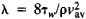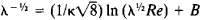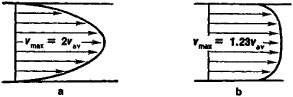# Turbulent Flow

Also found in: Dictionary, Thesaurus, Medical, Legal, Financial, Wikipedia.
Related to Turbulent Flow: laminar flow, turbulent blood flow

## Turbulent flow

A fluid motion in which velocity, pressure, and other flow quantities fluctuate irregularly in time and space. The illustration shows a slice of a water jet emerging from a circular orifice into a tank of still water. A small amount of fluorescent dye mixed in the jet makes it visible when suitably illuminated by laser light, and tags the water entering the tank. There is a small region close to the orifice where the dye concentration does not vary with position, or with time at a given position. This represents a steady laminar state. Generally in laminar motion, all variations of flow quantities, such as dye concentration, fluid velocity, and pressure, are smooth and gradual in time and space. Farther downstream, the jet undergoes a transition to a new state in which the eddy patterns are complex, and flow quantities (including vorticity) fluctuate randomly in time and three-dimensional space. This is the turbulent state. See Jet flow, Laminar flow

Turbulence occurs nearly everywhere in nature. It is characterized by the efficient dispersion and mixing of vorticity, heat, and contaminants. In flows over solid bodies such as airplane wings or turbine blades, or in confined flows through ducts and pipelines, turbulence is responsible for increased drag and heat transfer. Turbulence is therefore a subject of great engineering interest. On the other hand, as an example of collective interaction of many coupled degrees of freedom, it is also a subject at the forefront of classical physics. See Degree of freedom (mechanics), Diffusion, Heat transfer

The illustration demonstrates the principal issues associated with turbulent flows. The first is the mechanism (or mechanisms) responsible for transition from the steady laminar state to the turbulent state. A second issue concerns the description of fully developed turbulence typified by the complex state far downstream of the orifice. Finally, it is of technological importance to be able to alter the flow behavior to suit particular needs. Less is known about eddy motions on the scale of centimeters and millimeters than about atomic structure on the subnanometer scale, reflecting the complexity of the turbulence problem. See Navier-Stokes equation

#### Origin of turbulence

A central role in determining the state of fluid motion is played by the Reynolds number. In general, a given flow undergoes a succession of instabilities with increasing Reynolds number and, at some point, turbulence appears more or less abruptly. It has long been thought that the origin of turbulence can be understood by sequentially examining the instabilities. This sequence depends on the particular flow and, in many circumstances, is sensitive to a number of details. A careful analysis of the perturbed equations of motion has resulted in a good understanding of the first two instabilities in a variety of circumstances. See Reynolds number

#### Fully developed turbulence

Quite often in engineering, the detailed motion is not of interest, but only the long-time averages or means, such as the mean velocity in a boundary layer, the mean drag of an airplane or pressure loss in a pipeline, or the mean spread rate of a jet. It is therefore desirable to rewrite the Navier-Stokes equations for the mean motion. The basis for doing this is the Reynolds decomposition, which splits the overall motion into the time mean and fluctuations about the mean. These macroscopic fluctuations transport mass, momentum, and matter (in fact, by orders of magnitude more efficiently than molecular motion), and their overall effect is thus perceived to be in the form of additional transport or stress. This physical effect manifests itself as an additional stress (called the Reynolds stress) when the Navier-Stokes equations are rewritten for the mean motion (the Reynolds equations). The problem then is one of prescribing the Reynolds stress, which contains the unknown fluctuations in quadratic form. A property of turbulence is that the Reynolds stress terms are comparable to the other terms in the Reynolds equation, even when fluctuations are a small part of the overall motion. An equation for the Reynolds stress itself can be obtained by suitably manipulating the Navier-Stokes equations, but this contains third-order terms involving fluctuations, and an equation for third-order terms involves fourth-order quantities, and so forth. This is the closure problem in turbulence. The Navier-Stokes equations are themselves closed, but the presence of nonlinearity and the process of averaging result in nonclosure.

Given this situation, much of the progress in the field has been due to (1) exploratory experiments and numerical simulations of the Navier-Stokes equations at low Reynolds numbers; and (2) plausible hypotheses in conjunction with dimensional reasoning, scaling arguments, and their experimental verification.

#### Control of turbulent flows

Some typical objectives of flow control are the reduction of drag of an object such as an airplane wing, the suppression of combustion instabilities, and the suppression of vortex shedding behind bluff bodies. Interest in flow control has been stimulated by the discovery that some turbulent flows possess a certain degree of spatial coherence at large scales. Successful control has also been achieved through the reduction of the skin friction on a flat plate by making small longitudinal grooves, the so-called riblets, on the plate surface, imitating shark skin. See Fluid flow

## Turbulent Flow

a type of liquid or gas flow in which the liquid or gas elements move in a random, unsteady manner along complex trajectories. Such motion results in strong mixing between the layers of the moving liquid or gas (see). The turbulent flows that have been studied in the greatest detail include the following: flows in pipes, in channels, and in boundary layers around bodies immersed in a liquid or gas flow; free turbulent flows, or jets; the wakes behind bodies moving relative to a liquid or gas; and mixing zones between flows of differing velocity that are not separated by any solid walls.

Turbulent flows differ from the corresponding laminar flows in that the turbulent flows have a complex internal structure, a different distribution of the averaged velocity over a cross section of the flow, and different overall characteristics, that is, a different dependence on the Reynolds number Re of the flow rate, the resistance coefficient, and the average mean velocity over a cross section or the maximum velocity. The averaged velocity profile of a turbulent flow in a pipe or channel differs from the parabolic profile of the corresponding laminar flow in that the former is characterized by a faster increase in velocity at the walls and a smaller curvature in the central part of the flow (Figure 1). With the exception of a thin layer near the wall, the velocity profile is described by a logarithmic law; that is, the velocity is linearly dependent on the logarithm of the distance to the wall. The relationship between the resistance coefficient(where τw is the shearing stress at the wall, ρ is the density of the fluid, and vav is the fluid velocity averaged over a cross section of the flow) and Re is given by the equationwhere K and B are constants.Figure 1. Averaged velocity profile: (a) for a laminar flow, (b) for a turbulent flow

In contrast to laminar boundary layers, a turbulent boundary layer usually has a distinct edge that fluctuates randomly with time in the range 0.4δ–1.2δ, where δ is the distance from the wall at which the averaged velocity is 0.99v, and v is the velocity outside the boundary layer. The averaged velocity profile in the wall region of a turbulent boundary layer is described by a logarithmic law, but in the outer region the velocity increases with distance from the wall more rapidly than it would in accordance with a logarithmic law. In this case, the dependence of λ on Re has a form analogous to that indicated above.

Jets, wakes, and mixing zones are approximately self-similar. Self-similarity here means that in every cross section x = const of any of these turbulent flows, where the distance x from some initial cross section is not too small, there can be introduced length and velocity scales L(x) and v(x) such that the dimensionless statistical characteristics of the hydrodynamical fields (in particular, the averaged velocity profiles) obtained by using these scales will be identical in all cross sections.

In the case of free turbulent flows, the region of space occupied by an eddying turbulent flow has at every moment in time distinct but very irregular boundaries outside which the flow is irrotational. The turbulence mixing zone in this case is more extensive than in boundary layers.

## turbulent flow

[′tər·byə·lənt ′flō]
(fluid mechanics)
Motion of fluids in which local velocities and pressures fluctuate irregularly, in a random manner. Also known as turbulence.

## turbulent flow

The motion of a fluid in which local velocities and pressures fluctuate highly irregularly with time, in contrast to streamline flow.
References in periodicals archive ?
In this research work, numerical investigations have been carried out to understand the flow and heat transfer behavior of different nanofluids in a horizontal rectangular pipe under turbulent flow condition.
This value is a physical quantity which represents the size of the largest energy-containing eddies traveling in the turbulent flow.
On a contaminated wing, however, the roughness in the laminar region of an airfoil causes the point of separation between laminar and turbulent flow to move upstream from its normal position in a non-uniform and non-predictable way.
In this paper, we address the problem of comparing the influence of gravity on particle statistics for turbulent flow in channel and pipe with the same Karman number.
The 42-month project is related to further develop the algebraic structure-based turbulence model (ASBM), so that it could integrate into the process of planning planes and helicopters, which encounter erratic and turbulent flow of air, which has important impact on both flight stability and fuel consumption.
Previous investigations on the convective heat transfer enhancement of nanofluids have been reported as follows: Xuan and Li  have first time presented the empirical correlation for the estimation of Nusselt number in laminar and turbulent flow condition using nanofluids Cu particles.
Turbulent flow has been the kernel flow regime that the drag reduction researches have focused upon.
Turbulent flow results in a relatively larger airway resistance when compared with laminar flow and a much larger driving pressure is required to produce the same flow rate.
Turbulent flow over a wavy surface displays characteristics that are not found in flow over a flat surface, since further spatial variations of the velocity and pressure are induced .
They have also been able to measure how such turbulence builds over time by measuring how that turbulent flow of material fluctuates as it crosses or hits a boundary wall in small confined boxes within a lab.

Site: Follow: Share:
Open / Close